Every presidential candidate has a list of states they’re expected to win, but there are always states that are too close to call because they have similar numbers of registered voters for each of the two dominant political parties: Democrat and Republican. It’s in these “swing” states that candidates invest [...]

Anatomy of a swing state was published on SAS Voices by Mary OsborneSAS has always believed in the power of education, but in today’s data-driven economy, it’s more important than ever to ensure our students are introduced to data science at an early age. We as a company are focusing our resources on creating student experiences in data literacy, computer science and [...]The triangulation theorem for polygons says that every simple polygon can be triangulated. In fact, if the polygon has V vertices, you can decompose it into V-2 non-overlapping triangles. In this article, a "polygon" always means a simple polygon. Also, a "random point" means one that is drawn at random from the uniform distribution.

The triangularization of a polygon is useful in many ways, but one application is to generate uniform random points in a polygon or a collection of polygons. Because polygons can be decomposed into triangles, the problem reduces to a simpler one: Given a list of k triangles, generate uniform random points in the union of the triangles. I have already shown how to generate random points in a triangle, so you can apply this method to generate random points in a polygon or collection of polygons.

### An algorithm to generate random points in a polygon

Suppose that a polygon or any other planar region is decomposed into k triangles T1, T2, ..., Tk. If you want to generate N random points uniformly in the region, the number of points in any triangle should be proportional to the area of the triangle divided by the total area of the polygon.

One way to accomplish this is to use a two-step process. First, choose a triangle by using a probability proportional to the relative areas. Next, generate a random point in that triangle. This two-step approach is suitable for the SAS DATA step. At the end of this process, you have generated Ni observations in triangle Ti.

An equivalent formulation is to realize that the vector {N1, N2, ..., Nk} is a random draw from the multinomial distribution with parameters p = {p1, p2, ..., pk}, where pi = Area(Ti) / (Σj Area(Tj)). This second formulation is better for a vector languages such as the SAS/IML language.

Therefore, the following algorithm generates random points in a polygon:

1. Decompose the polygon into triangles T1, T2, ..., Tk.
2. Compute the areas: Ai = Area(Ti).
3. Generate one random draw from the multinomial distribution with probability vector p = {p1, p2, ..., pk}, where pi = Ai / (Σj Aj). This gives a vector of numbers {N1, N2, ..., Nk}.
4. Use the algorithm from the previous article to generate Ni random points in the triangle Ti.

Notice that Steps 2-4 of this algorithm apply to ANY collection of triangles. To make the algorithm flexible, I will implement the first step (the decomposition) in one function and the remaining steps in a second function.

### Triangulate a convex polygon in SAS

There are various general methods for triangulating a polygon, but for convex polygons, there is a simple method. From among the V vertices, choose any vertex and call it P1. Enumerate the remaining vertices consecutively in a counter-clockwise direction: P2, P3, ..., Pk, where k = V-2. Because the polygon is convex, the following triangles decompose the polygon:

• T1 = {P1, P2, P3}
• T2 = {P1, P3, P4}, and so forth, up to
• Tk-2 = {P1, Pk-1, Pk}

The following SAS/IML function decomposes a convex polygon into triangles. The triangles are returned in a SAS/IML list. The function is called on a convex hexagon and the resulting decomposition is shown below. The function uses the PolyIsConvex function, which is part of the Polygon package. You can download and install the Polygon package. You need to load the Polygon package before you call the function.

```/* assume the polygon package is installed */ proc iml; package load polygon; /* load the polygon package */   /* Decompose a convex polygon into triangles. Return a list that contains the vertices for the triangles. This function uses a function in the Polygon package, which must be loaded. */ start TriangulateConvex(P); /* input parameter(N x 2): vertices of polygon */ isConvex = PolyIsConvex(P); if ^isConvex then return ( [] ); /* The polygon is not convex */ numTri = nrow(P) - 2; /* number of triangles in convex polygon */ L = ListCreate(numTri); /* create list to store triangles */ idx = 2:3; do i = 1 to ListLen(L); L\$i = P[1,] // P[idx,]; idx = idx + 1; end; return (L); finish;   /* Specify a convex polygon and visualize the triangulation. */ P = { 2 1 , 3 1 , 4 2 , 5 4 , 3 6 , 1 4 , 1 2 }; L = TriangulateConvex(P);```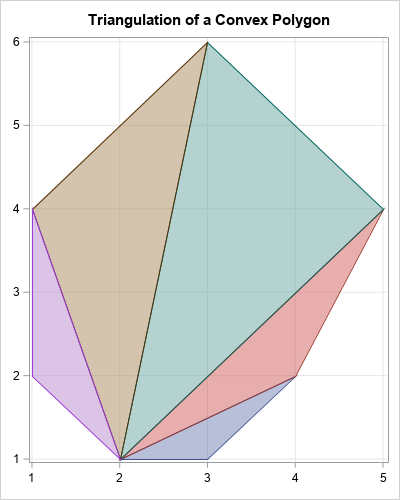To illustrate the process, I've included a graph that shows a decomposition of the convex hexagon into triangles. The triangles are returned in a list. The next section shows how to generate uniform points at random inside the union of the triangles in this list.

### Generate random points in a collection of triangles

This section generates random points in a union of triangles. The following function takes two arguments: the number of points to generate (N) and a list of triangles (L). The algorithm computes the relative areas of the triangles and uses them to determine the probability that a point will be generated in each. It then uses the RandUnifTriangle function from the previous article to generate the random points.

```/* Given a list of triangles (L), generate N random points in the union, where the number of points is proportional to Area(triangle) / Area(all triangles)   This function uses functions in the Polygon package, which must be loaded. */ start RandUnifManyTriangles(N, L); numTri = ListLen(L); /* compute areas of each triangle in the list */ AreaTri = j(1, numTri,.); /* create vector to store areas */ do i = 1 to numTri; AreaTri[i] = PolyArea(L\$i); /* PolyArea is in the Polygon package */ end; /* Numbers of points in the triangles are multinomial with probability proportional to Area(triangle)/Area(polygon) */ NTri = RandMultinomial(1, N, AreaTri/sum(AreaTri)); cumulN = 0 || cusum(NTri); /* cumulative counts; use as indices */ z = j(N, 3, .); /* columns are (x,y,TriangleID) */ do i = 1 to numTri; k = (cumulN[i]+1):cumulN[i+1]; /* the next NTri[i] elements */ z[k, 1:2] = RandUnifTriangle(L\$i, NTri[i]); z[k, 3] = i; /* store the triangle ID */ end; return z; finish;   /* The RandUnifTriangle function is defined at https://blogs.sas.com/content/iml/2020/10/19/random-points-in-triangle.html */ load module=(RandUnifTriangle); call randseed(12345); N = 2000; z = RandUnifManyTriangles(N, L);```

The z vector is an N x 3 matrix. The first two columns contain the (x,y) coordinates of N random points. The third column contains the ID number (values 1,2,...,k) that indicates the triangle that each point is inside of. You can use the PolyDraw function in the Polygon package to visualize the distribution of the points within the polygon:

```title "Random Points in a Polygon"; title2 "Colors Assigned Based on Triangulation"; call PolyDraw(P, z);```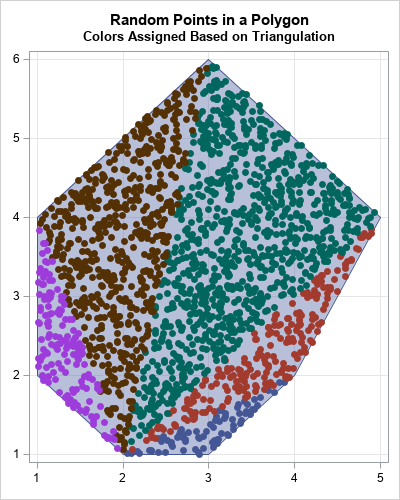The color of each point indicates which triangle the point is inside. You can see that triangles with relatively small areas (blue and purple) have fewer points than triangles with larger areas (green and brown).

### Summary

In summary, this article shows how to generate random points inside a planar polygon. The first step is to decompose the polygon into triangles. You can use the relative areas of the triangles to determine the probability that a random point is in each triangle. Finally, you can generate random points in the union of the triangles. (Note: The algorithm works for any collection of planar triangles.)

This article uses functions in the Polygon package. Installing and loading a package is a way to define a set of related functions that you want to share. It is an alternative to using %INCLUDE to include the module definitions into your program.

The post Generate random points in a polygon appeared first on The DO Loop.The triangulation theorem for polygons says that every simple polygon can be triangulated. In fact, if the polygon has V vertices, you can decompose it into V-2 non-overlapping triangles. In this article, a "polygon" always means a simple polygon. Also, a "random point" means one that is drawn at random from the uniform distribution.

The triangularization of a polygon is useful in many ways, but one application is to generate uniform random points in a polygon or a collection of polygons. Because polygons can be decomposed into triangles, the problem reduces to a simpler one: Given a list of k triangles, generate uniform random points in the union of the triangles. I have already shown how to generate random points in a triangle, so you can apply this method to generate random points in a polygon or collection of polygons.

### An algorithm to generate random points in a polygon

Suppose that a polygon or any other planar region is decomposed into k triangles T1, T2, ..., Tk. If you want to generate N random points uniformly in the region, the number of points in any triangle should be proportional to the area of the triangle divided by the total area of the polygon.

One way to accomplish this is to use a two-step process. First, choose a triangle by using a probability proportional to the relative areas. Next, generate a random point in that triangle. This two-step approach is suitable for the SAS DATA step. At the end of this process, you have generated Ni observations in triangle Ti.

An equivalent formulation is to realize that the vector {N1, N2, ..., Nk} is a random draw from the multinomial distribution with parameters p = {p1, p2, ..., pk}, where pi = Area(Ti) / (Σj Area(Tj)). This second formulation is better for a vector languages such as the SAS/IML language.

Therefore, the following algorithm generates random points in a polygon:

1. Decompose the polygon into triangles T1, T2, ..., Tk.
2. Compute the areas: Ai = Area(Ti).
3. Generate one random draw from the multinomial distribution with probability vector p = {p1, p2, ..., pk}, where pi = Ai / (Σj Aj). This gives a vector of numbers {N1, N2, ..., Nk}.
4. Use the algorithm from the previous article to generate Ni random points in the triangle Ti.

Notice that Steps 2-4 of this algorithm apply to ANY collection of triangles. To make the algorithm flexible, I will implement the first step (the decomposition) in one function and the remaining steps in a second function.

### Triangulate a convex polygon in SAS

There are various general methods for triangulating a polygon, but for convex polygons, there is a simple method. From among the V vertices, choose any vertex and call it P1. Enumerate the remaining vertices consecutively in a counter-clockwise direction: P2, P3, ..., Pk, where k = V-2. Because the polygon is convex, the following triangles decompose the polygon:

• T1 = {P1, P2, P3}
• T2 = {P1, P3, P4}, and so forth, up to
• Tk-2 = {P1, Pk-1, Pk}

The following SAS/IML function decomposes a convex polygon into triangles. The triangles are returned in a SAS/IML list. The function is called on a convex hexagon and the resulting decomposition is shown below. The function uses the PolyIsConvex function, which is part of the Polygon package. You can download and install the Polygon package. You need to load the Polygon package before you call the function.

```/* assume the polygon package is installed */ proc iml; package load polygon; /* load the polygon package */   /* Decompose a convex polygon into triangles. Return a list that contains the vertices for the triangles. This function uses a function in the Polygon package, which must be loaded. */ start TriangulateConvex(P); /* input parameter(N x 2): vertices of polygon */ isConvex = PolyIsConvex(P); if ^isConvex then return ( [] ); /* The polygon is not convex */ numTri = nrow(P) - 2; /* number of triangles in convex polygon */ L = ListCreate(numTri); /* create list to store triangles */ idx = 2:3; do i = 1 to ListLen(L); L\$i = P[1,] // P[idx,]; idx = idx + 1; end; return (L); finish;   /* Specify a convex polygon and visualize the triangulation. */ P = { 2 1 , 3 1 , 4 2 , 5 4 , 3 6 , 1 4 , 1 2 }; L = TriangulateConvex(P);```To illustrate the process, I've included a graph that shows a decomposition of the convex hexagon into triangles. The triangles are returned in a list. The next section shows how to generate uniform points at random inside the union of the triangles in this list.

### Generate random points in a collection of triangles

This section generates random points in a union of triangles. The following function takes two arguments: the number of points to generate (N) and a list of triangles (L). The algorithm computes the relative areas of the triangles and uses them to determine the probability that a point will be generated in each. It then uses the RandUnifTriangle function from the previous article to generate the random points.

```/* Given a list of triangles (L), generate N random points in the union, where the number of points is proportional to Area(triangle) / Area(all triangles)   This function uses functions in the Polygon package, which must be loaded. */ start RandUnifManyTriangles(N, L); numTri = ListLen(L); /* compute areas of each triangle in the list */ AreaTri = j(1, numTri,.); /* create vector to store areas */ do i = 1 to numTri; AreaTri[i] = PolyArea(L\$i); /* PolyArea is in the Polygon package */ end; /* Numbers of points in the triangles are multinomial with probability proportional to Area(triangle)/Area(polygon) */ NTri = RandMultinomial(1, N, AreaTri/sum(AreaTri)); cumulN = 0 || cusum(NTri); /* cumulative counts; use as indices */ z = j(N, 3, .); /* columns are (x,y,TriangleID) */ do i = 1 to numTri; k = (cumulN[i]+1):cumulN[i+1]; /* the next NTri[i] elements */ z[k, 1:2] = RandUnifTriangle(L\$i, NTri[i]); z[k, 3] = i; /* store the triangle ID */ end; return z; finish;   /* The RandUnifTriangle function is defined at https://blogs.sas.com/content/iml/2020/10/19/random-points-in-triangle.html */ load module=(RandUnifTriangle); call randseed(12345); N = 2000; z = RandUnifManyTriangles(N, L);```

The z vector is an N x 3 matrix. The first two columns contain the (x,y) coordinates of N random points. The third column contains the ID number (values 1,2,...,k) that indicates the triangle that each point is inside of. You can use the PolyDraw function in the Polygon package to visualize the distribution of the points within the polygon:

```title "Random Points in a Polygon"; title2 "Colors Assigned Based on Triangulation"; call PolyDraw(P, z);```The color of each point indicates which triangle the point is inside. You can see that triangles with relatively small areas (blue and purple) have fewer points than triangles with larger areas (green and brown).

### Summary

In summary, this article shows how to generate random points inside a planar polygon. The first step is to decompose the polygon into triangles. You can use the relative areas of the triangles to determine the probability that a random point is in each triangle. Finally, you can generate random points in the union of the triangles. (Note: The algorithm works for any collection of planar triangles.)

This article uses functions in the Polygon package. Installing and loading a package is a way to define a set of related functions that you want to share. It is an alternative to using %INCLUDE to include the module definitions into your program.

The post Generate random points in a polygon appeared first on The DO Loop.Who Helps the World? Girls! Decades of research show there's one strong difference in what young men and women say they value in a future career: The opportunity to help others. And young women are far more likely to say that they want a job where they can have a positive [...]

An open letter to girls: It's time to dream bigger was published on SAS Voices by Jen SabourinWhen you use SAS software, you might occasionally encounter an issue with SASUSER. This post helps you debug some of the more common issues:

• a warning message indicates that SASUSER.TEMPLAT is not an item store or that you cannot write to SASUSER.TEMPLAT
• a note in the log indicates that SAS cannot open the SASUSER.PROFILE catalog
• a note in the log indicates that SAS cannot open the SASUSER.REGSTRY item store
• various errors and abnormal endings occur when you use the SAS® Output Delivery System or create graphics output

## Issue 1: SAS® writes a message to the log indicating that SASUSER.TEMPLAT is not an item store or that you cannot write to SASUSER.TEMPLAT

By default, SAS tries to store custom templates and styles that PROC TEMPLATE creates in SASUSER. In some SAS environments with multiple users on a server, your SASUSER location might be read-only (set with the RSASUSER option). If you do not need the template or style to persist between sessions, you can set the template path to include the WORK library first:

```ods path(prepend) work.template(update);
```

If you are working with a local SAS session, this issue can occur when a corrupt or old copy of the templat.sas7bitm file exists in your SASUSER directory. To resolve the issue

1. Determine the location of your SASUSER directory by submitting the following code to SAS:
```proc options option=sasuser; run;```
1. View the new information that is written to the log and make a note of the directory to which SASUSER points.
2. Stop all running SAS sessions.
3. From your operating system, open your SASUSER directory and rename templat.sas7bitm to templat.old.
4. Restart SAS.

## Issue 2: SAS® writes a note or warning to the log indicating that SAS cannot open SASUSER.PROFILE

If you see a note or warning in the log indicating that SAS cannot open the SASUSER.PROFILE catalog, you should ensure first that you have only a single SAS session running.  If you have multiple SAS sessions running concurrently only the first SAS session has Update access to SASUSER.

If only one SAS session is active and you still receive a note or warning that SAS cannot open SASUSER.PROFILE:

1. Determine the location of your SASUSER directory by submitting the following code to SAS:
```proc options option=sasuser; run;```
1. Stop any running SAS sessions.
2. Rename the following files in your SASUSER directory:

In Microsoft Windows operating environments, rename the files as follows:

• profile.sas7bcat to profile.old
• profbak.sas7bat to profbak.old
• profile2sas7bcat to profile2.old

In UNIX operating environments, rename the files as follows:

• profile.sas7bcat to profile.old
• profile.sas7bcat to profbak.old

## Issue 3: SAS® writes a note or warning to the log indicating that SAS cannot open the SASUSER.REGSTRY item store

If you see a note or warning in the log indicating that SAS cannot open SASUSER.REGSTRY, ensure first that you have only a single SAS session running. If you have multiple SAS sessions running concurrently only the first SAS session has Update access to SASUSER.

If only one SAS session is active and you still receive a note or warning that SAS cannot open SASUSER.REGSTRY:

1. Determine the location of your SASUSER directory by submitting the following code to SAS:
```proc options option=sasuser; run;```
1. Stop any running SAS sessions.
2. From your operating environment, open your SASUSER directory and rename regstry.sas7bitm to regstry.old.
3. Restart SAS.

## Issue 4: Various abnormal endings and errors occur when you use SAS Output Delivery System (ODS) or when you create graphics output

If one or more files or catalogs in SASUSER are corrupted, various abnormal endings and errors can occur when you use ODS or when you create graphics output.

If you suspect that this is the case, determine the location of your SASUSER directory by submitting the following code to SAS:

```proc options option=sasuser; run;```
1. View the new information that is written to the log and make a note of the directory to which SASUSER points.
2. Stop all running SAS sessions.
3. From your operating environment, open the SASUSER directory and rename the following files (if they exist) as shown:
• profile2.sas7bcat to profile2.old
• regstry.sas7bitm to regstry.old
• templat.sas7bitm to templat.old
1. Restart SAS.

If you follow the debugging steps for any of the issues outlined above and find that you still have Read access to SASUSER, the problem might be with your SAS installation. Specifically, your installation

 ```proc options option=rsasuser; run;```

In a multiuser SAS environment or SAS Grid Computing environment, RSASUSER might be set by policy. In that case, you must adjust your programs/process to not rely on SASUSER for personal content. If working with a local or private SAS environment, you can change the option to NORSASUSER in your SAS configuration file.

## Summary

As you can see from this post, a variety of reasons can cause issues with the SASUSER directory. These issues can occur when one or more catalogs or item stores in your SASUSER directory become corrupted or are created with an earlier installation of SAS. However, if you rename the catalogs or item stores with a file extension that SAS does not recognize, SAS creates new, uncorrupted copies of these files when you restart SAS.

Debugging SASUSER issues when you use SAS® software was published on SAS Users.Depending on who you talk to, you'll get varying definitions and opinions regarding demand sensing. Anything from sensing short-range replenishment based on sales orders, to the manual blending of point-of-sales (POS) data and shipments. But a key component for retailers and CPG companies is accurately forecasting short-term consumer demand to [...]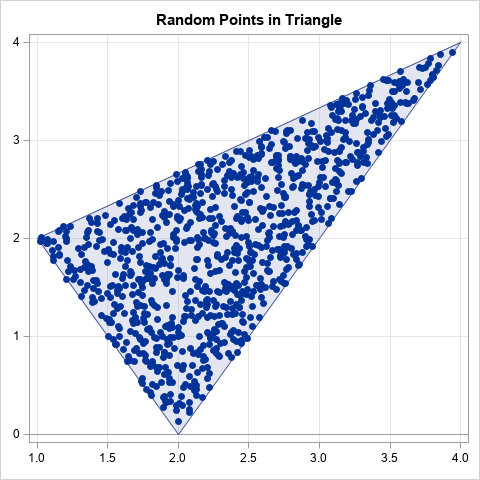How can you efficiently generate N random uniform points in a triangular region of the plane? There is a very cool algorithm (which I call the reflection method) that makes the process easy. I no longer remember where I saw this algorithm, but it is different from the "weighted average" method in Devroye (1986, p. 569-570). This article describes and implements the reflection algorithm for generating random points in a triangle from the uniform distribution. The graph to the right shows 1,000 random points in the triangle with vertices P1=(1, 2), P2=(4, 4), and P3=(2, 0). The method works for any kind of triangle: acute, obtuse, equilateral, and so forth.

In this article, "random points" means that the points are drawn randomly from the uniform distribution.

### Random points in a parallelogram

The easiest way to understand the algorithm is to think about generating points in a parallelogram. For simplicity, translate the parallelogram so that one vertex is at the origin. Two sides of the parallelogram share that vertex. Let a and b be the vectors from the origin to the adjacent vertices.

To produce a random point in the parallelogram, generate u1, u2 ~ U(0,1) and form the vector sum
p = u1*a + u2*b
This is the 2-D parameterization of the parallelogram, so for random u1 and u2, the point p is uniformly distributed in the parallelogram. The geometry is shown in the following figure.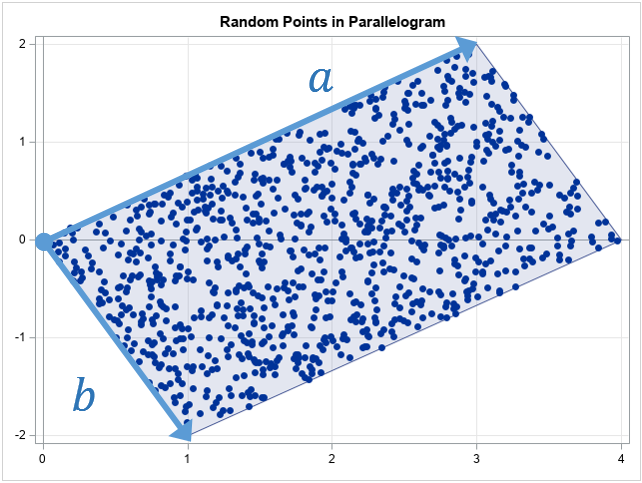The following SAS/IML program generates 1,000 random points in the parallelogram. The graph is shown above.

```proc iml; n = 1000; call randseed(1234);   /* random points in a parallelgram */ a = {3 2}; /* vector along one side */ b = {1 -2}; /* vector along adjacent side */ u = randfun(n // 2, "Uniform"); /* u[,1], u[,2] ~ U(0,1) */ w = u[,1]@a + u[,2]@b; /* linear combination of a and b */ title "Random Points in Parallelogram"; call scatter(w[,1], w[,2]) grid={x,y};```

The only mysterious part of the program is the use of the Kronecker product (the '@' operator) to form linear combinations of the a and b vectors. The details of the Kronecker product operator are described in a separate article. Briefly, it is an efficient way to generate linear combinations of a and b without writing a loop.

### The reflection step

A useful fact about random uniform variates is that if u ~ U(0,1), then also v = 1 - u ~ U(0,1). You can use this fact to convert N points in a parallelogram into N points in a triangle.

Let u1, u2 ~ U(0,1) be random variates in (0,1). If u1 + u2 ≤ 1, then the vector u1*a + u2*b is in the triangle with sides a and b. If u1 + u2 > 1, then define v1 = 1 - u1 and v2 = 1 - u2, which are also random uniform variates. You can verify that the sum v1 + v2 ≤ 1, which means that the vector v1*a + v2*b is in the triangle with sides a and b.

This is shown in the following graph. The blue points are the points for which u1 + u2 ≤ 1. The red points are for u1 + u2 > 1. When you form v1 and v2, the red triangle get reflected twice and ends up on top of the blue triangle. The two reflections are equivalent to a 180 degree rotation about the center of the parallelogram, which might be easier to visualize.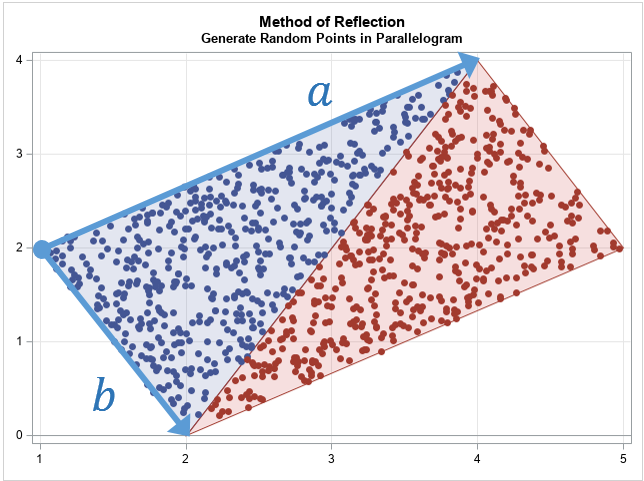### Generate random points in a triangle

With this background, you can now generate random points in any triangle. Let P1, P2, and P3 be the vertices of the triangle. The algorithm to generate random points in the triangle is as follows:

1. Define the vectors a = P2 - P1 and b = P3 - P1. The vectors define the sides of the triangle when it is translated to the origin.
2. Generate random uniform values u1, u2 ~ U(0,1)
3. If u1 + u2 > 1, apply the transformation u1 → 1 - u1 and u2 → 1 - u2.
4. Form w = u1 a + u2 b, which is a random point in the triangle at the origin.
5. The point w + P1 is a random point in the original triangle.

The following SAS/IML program implements this algorithm and runs it for the triangle with vertices P1=(1, 2), P2=(4, 4), and P3=(2, 0).

```/* generate random uniform sample in triangle with vertices P1 = (x0,y0), P2 = (x1,y1), and P3 = (x2,y2) The triangle is specified as a 3x2 matrix, where each row is a vertex. */ start randUnifTriangle(P, n); a = P[2,] - P[1,]; /* translate triangle to origin */ b = P[3,] - P[1,]; /* a and b are vectors at the origin */ u = randfun(n // 2, "Uniform"); idx = loc(u[,+] >= 1); /* identify points outside of the triangle */ if ncol(idx)>0 then u[idx,] = 1 - u[idx,]; /* transform variates into the triangle */ w = u[,1]@a + u[,2]@b; /* linear combination of a and b vectors */ return( P[1,] + w ); /* translate triangle back to original position */ finish; store module=(randUnifTriangle);   /* triangle contains three vertices */ call randseed(1234,1); P = {1 2, /* P1 */ 4 4, /* P2 */ 2 0}; /* P3 */ n = 1000; w = randUnifTriangle(P, n);   title "Random Points in Triangle"; ods graphics / width=480px height=480px; call scatter(w[,1], w[,2]) grid={x,y};```

The graph of the 1,000 random points appears at the top of this program.

### Showing scatter plots with polygons

As written, the programs in this article create scatter plots that show the random points. To improve the exposition, I used the polygon package to draw graphs that overlay the scatter plot and a polygon. You can download and install the polygon package if you have PROC IML with SAS 9.4m3 or later. You can download the complete SAS program that performs all the computations and creates all the graphs in this article.

### Summary

In summary, this article shows how to generate random uniform points in a triangle by using the reflection algorithm. The reflection algorithm is based on generating random points in a parallelogram. If you draw the diagonal of a parallelogram, you get two congruent triangles. The algorithm reflects (twice) all points in one triangle into the other triangle. The algorithm is implemented in SAS by using the SAS/IML language, although you could also use the SAS DATA step.

The post Generate random points in a triangle appeared first on The DO Loop.Two billion people worldwide experience food insecurity and 14% of food produced for humans to consume is wasted before it arrives at the wholesaler, according to the Food and Agriculture Organization of the United Nations (FAO). The FAO celebrates World Food Day on October 16, 2020. This year’s event focuses [...]